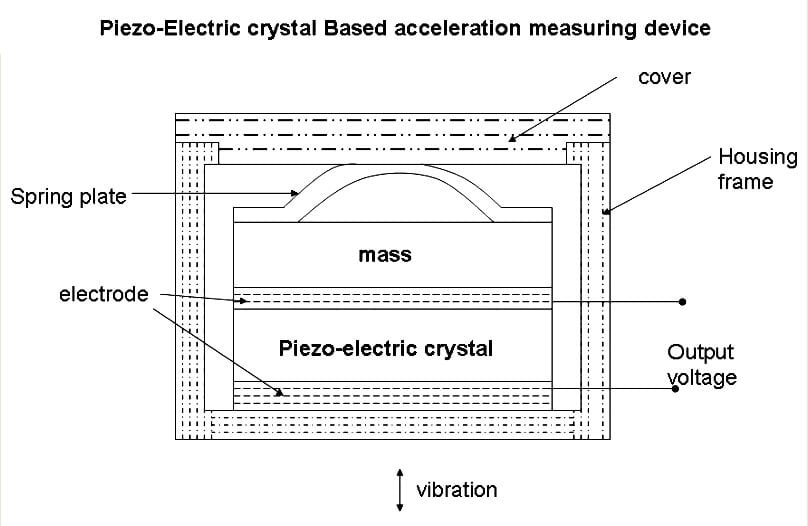# Piezo-electric Acceleration Measuring Device

Piezo-electric Crystal based Acceleration measuring device

Piezo Electric Concept:

This device which you will build is based on piezo electric effect. Let me explain you in detail, when a piezo-electric crystal is subjected to a mechanical force or stresses along specific planes, a voltage is generated across the crystal. If the force on the crystal is due to acceleration, a measure of the voltage across the crystal becomes a measure of the acceleration when calibrated.

Designing the Device:You can see the image above that gives a very clear sketch of the device that is to be built, a piezo-electric crystal is placed between two electrodes and a mass is placed just above the electrode-crystal combination as shown in the above image. The crystal is should be loaded using a spring plate so that the crystal moves whenever there is a vibration or acceleration. This entire setup crystal, electrode, mass and the spring plate arrangement is placed inside a housing unit.

How it operates:

Now the Device is ready for working after connecting all the components and the device is fitted on the structure whose acceleration is to be measured. The structure accelerates and due to the acceleration the spring presses the mass and a fore is exerted on the piezo-electric crystal.

Its time to be theoretical,

We know Force = mass*acceleration.

As the mass is a constant, the generated force is proportional to the acceleration. Due to the force, a voltage is generated across the crystal, which is picked up by the electrodes which are fitted across the crystal. This voltage becomes a measure of acceleration when calibrated.